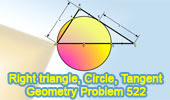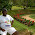## Sunday, September 26, 2010

### Problem 522: Right Triangle, Circle, Diameter, Tangent

Geometry Problem
Level: High School, SAT Prep, College geometry.
Click the figure below to see the complete problem 522 about Right Triangle, Circle, Diameter, Tangent.1.▲ADE ~ ▲ABE => ED/AE = BE/x
▲EDC ~ ▲AEC => ED/AE = DC/5
=> x = 5

2.http://ahmetelmas-geo-geo-antonio.blogspot.com/2010/09/prb522.html

3.Because FD // BC, FE = DE = m say

If also BE = DC = p and AE = n

p/m = x/n = 5/n since Tr.s BEF and ABE are similar and DEC and ACE are similar

So x = 5

Sumith Peiris
Moratuwa
Sri Lanka

4.Solution 2

FD//BC, ODEC is a kite as before.

So AE bisects < BAC

So x/BE = AC/5 or x = AC.BE/5 = CD.AC/5 = 25/5 = 5

Sumith Peiris
Moratuwa
Sri Lanka